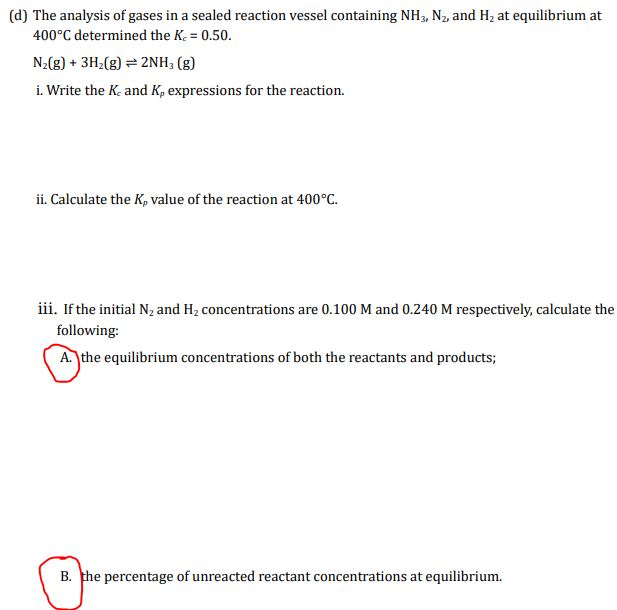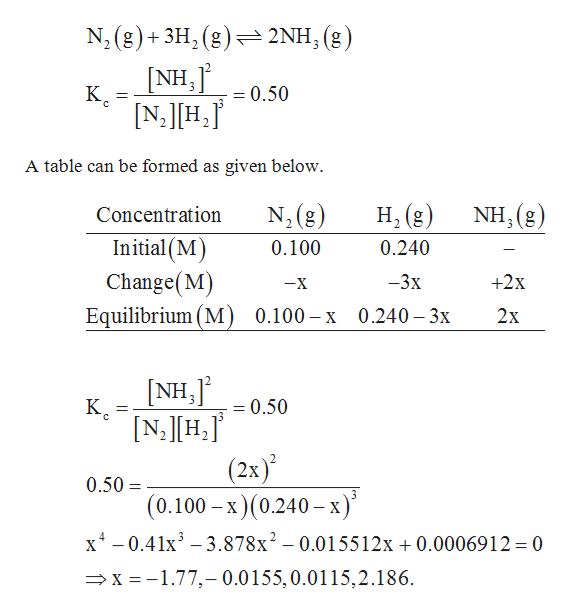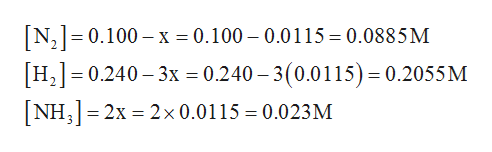# (d) The analysis of gases in a sealed reaction vessel containing NH3, N2, and H2 at equilibrium at400°C determined the Ke 0.50No(g)+3H2(g) 2NH3 (g)i. Write the Ke and K, expressions for the reaction.ii. Calculate the K, value of the reaction at 400°Ciii.If the initial N2 and H2 concentrations are 0.100 M and 0.240 M respectively, calculate thefollowing:A. the equilibrium concentrations of both the reactants and products;B. the percentage of unreacted reactant concentrations at equilibrium.

Question
47 views

Questions d(a) and d(b). I have completed other parts.help_outlineImage Transcriptionclose(d) The analysis of gases in a sealed reaction vessel containing NH3, N2, and H2 at equilibrium at 400°C determined the Ke 0.50 No(g)+3H2(g) 2NH3 (g) i. Write the Ke and K, expressions for the reaction. ii. Calculate the K, value of the reaction at 400°C iii.If the initial N2 and H2 concentrations are 0.100 M and 0.240 M respectively, calculate the following: A. the equilibrium concentrations of both the reactants and products; B. the percentage of unreacted reactant concentrations at equilibrium. fullscreen
check_circle

star
star
star
star
star
1 Rating
Step 1
1. The balanced chemical reaction is given below.help_outlineImage TranscriptioncloseN, (g)3H2(g)2NH (g NH,j 3 К. = 0.50 N,H, A table can be formed as given below Н (g) ) NH, (g) N2(g Concentration 3 Initial (M 0.100 0.240 Change(M) Equilibrium (M) 0.100-х 0.240-3х -3x +2x -X 2х NH,J К. [N,H, = 0.50 (2x) 0.50 = (0.100- х) (0.240—х)* x-0.41x3 3.878x2- 0.015512x 0.0006912 = 0 x 1.77,- 0.0155,0.0115,2.186 fullscreen
Step 2

The value of x is 0.0115 should be taken as negative values can not be taken and 2.186 is not logically suitable as it will give negative a...help_outlineImage TranscriptioncloseN2 0.100 x 0.100 - 0.0115 0.0885M H, 0.240 3x 0.240 3(0.0115)= 0.2055 M NH3 2x 2x 0.0115 = 0.023M fullscreen

### Want to see the full answer?

See Solution

#### Want to see this answer and more?

Solutions are written by subject experts who are available 24/7. Questions are typically answered within 1 hour.*

See Solution
*Response times may vary by subject and question.
Tagged in

### Chemistry